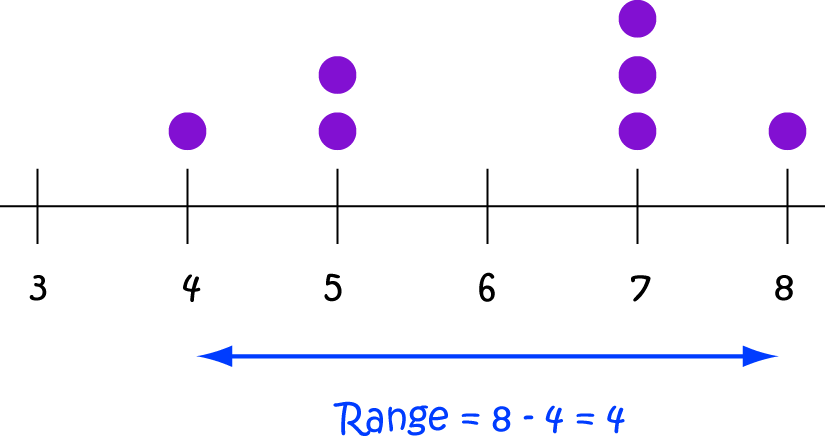# Definition of Range (Statistics)The range of a data set is the difference between its highest and lowest values.

For example, the lowest value of the data set $\{2,3,7,9,1,4\}$ is $1$ and its highest value is $9$, so its range is $9 - 1 = 8$.

The highest value on the dot plot is $8$ and the lowest value is $4$, so the range of the data set represented by the dot plot is $8 - 4 = 4$.

### Description

The aim of this dictionary is to provide definitions to common mathematical terms. Students learn a new math skill every week at school, sometimes just before they start a new skill, if they want to look at what a specific term means, this is where this dictionary will become handy and a go-to guide for a student.

### Audience

Year 1 to Year 12 students

### Learning Objectives

Learn common math terms starting with letter R

Author: Subject Coach
You must be logged in as Student to ask a Question.